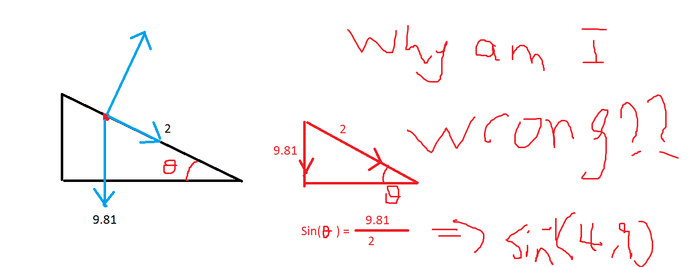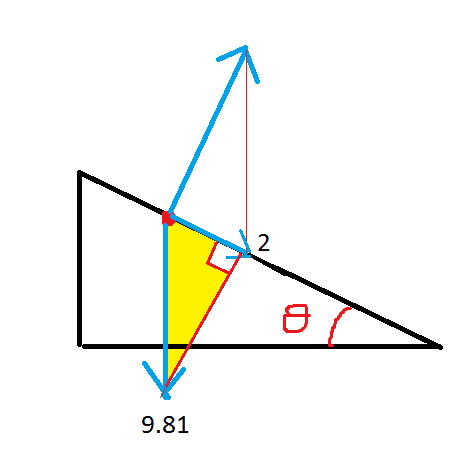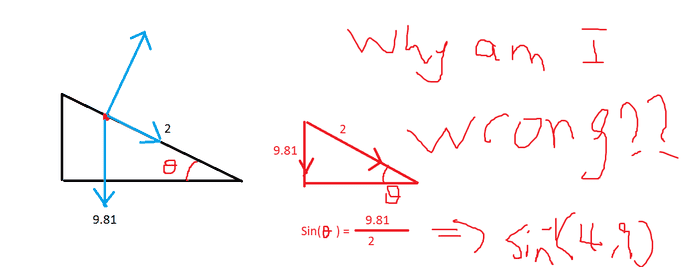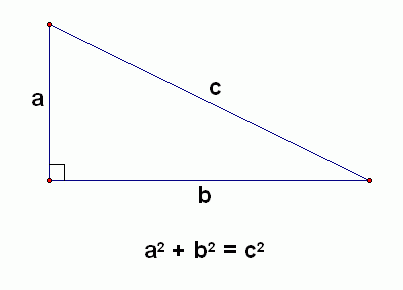# Why is this triangle not right? (sine -1 is bigger than 1)

• 1832vin
In summary, your black triangle is given and your red triangle is wrong because you have not correctly related the vectors in the diagram to each other.

#### 1832vinthe black triangle is given, and red triangle is me trying to work it out
why is my trangle wrong? and what is the right triangle?
someone is sliding down a slope, with 2ms-2 of acceleration (not actually given which direction, maybe I'm wrong there, but the person is acelerating at 2ms-2 down the slope)

why is my triangle wrong? can some one please draw me a right triangle...
i can't believe I'm stuck on this...

1832vin said:the black triangle is given, and red triangle is me trying to work it out
why is my trangle wrong? and what is the right triangle?
someone is sliding down a slope, with 2ms-2 of acceleration (not actually given which direction, maybe I'm wrong there, but the person is acelerating at 2ms-2 down the slope)

why is my triangle wrong? can some one please draw me a right triangle...
i can't believe I'm stuck on this...
What do the vectors represent?

How should they be related to each other?

What is the problem you're trying to solve?

Try ## \sin \theta=2/9.81 ##. (Your second triangle is incorrect).

Try ## \sin \theta=2/9.81 ##. (Your second triangle is incorrect).
yes, i know that'd be the right answer

but why have i got it wrong? thankyou

1832vin said:
yes, i know that'd be the right answer

but why have i got it wrong? thankyou
The downward gravitational force is ## F_g=mg ##. This can be broken into two components, one parallel to the plane and one perpendicular. The perpendicular component gets canceled by the force the plane exerts on the object. All acceleration is along the plane. You should be able to figure out the remaining part.

The 90° angle is opposite to the side of length 9.81. It is the hypotenuse of the yellow right triangle. In your drawing, the right triangle is opposite the shorter side. In every triangle, a side opposite to a smaller angle is shorter than a side opposite to a greater angle. If one angle is 90° in a triangle, the other angles must be smaller, as the sum of the angles can not exceed 180°. So the side opposite to a right angle is the longest side of the triangle.1832vin said:the black triangle is given, and red triangle is me trying to work it out
why is my trangle wrong? and what is the right triangle?
someone is sliding down a slope, with 2ms-2 of acceleration (not actually given which direction, maybe I'm wrong there, but the person is acelerating at 2ms-2 down the slope)

why is my triangle wrong? can some one please draw me a right triangle...
i can't believe I'm stuck on this...

Your red triangle is an impossible geometric figure: it is a right-triangle with a hypotenuse of 2 but a side of 9.81! Every right-triangle has a hypotenuse as large as each side (and strictly larger than both if it has not collapsed down to a straight-line segment).

@1832vin ,

Did you check to see what a calculator gives you for ##\ \sin^{-1}(4.9) \ ?##

1832vin said:the black triangle is given, and red triangle is me trying to work it out
why is my trangle wrong? and what is the right triangle?
someone is sliding down a slope, with 2ms-2 of acceleration (not actually given which direction, maybe I'm wrong there, but the person is acelerating at 2ms-2 down the slope)

why is my triangle wrong? can some one please draw me a right triangle...
i can't believe I'm stuck on this...
The Pythagorean relation is central to understanding right triangles and the trig functions derived from them.

You should have this diagram memorized:This should avoid making silly mistakes like the triangle you drew originally.1832vin said:
why is my triangle wrong
Your black triangle shows three vectors as blue arrows. The red triangle you obtain from that should have sides parallel to those three vectors. You have one parallel to the vertical blue arrow and one parallel to the downslope blue arrow, but your third is horizontal instead of normal to the slope.